### Home > INT3 > Chapter 7 > Lesson 7.1.4 > Problem7-48

7-48.
1. What is the inverse of each of the functions below? Write your answers using function notation. Homework Help ✎

1. p(x) = 3(x3 + 6)

2. k(x) = 3x3 + 6

3. h(x) =4. j(x) =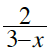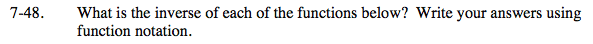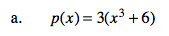Let p(x) = y, switch the x and the y in the equation and solve for y.

$p^{-1}(x)=\sqrt{\frac{x}{3}-6}$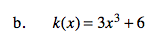See part (a).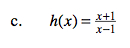Let h(x) = y . Switch x and y. Multiply both sides of the equation by (y − 1) to remove the fraction.

Distribute and then rewrite the equation so that all the y-terms are on the left side and everything else is on the right side.

Your equation should look like this: xyy = x + 1. Factor the left side of the equation and divide both sides by (x − 1) to get y by itself.

Graph the equation on a graphing calculator to see why this equation is its own inverse.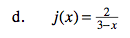See part (a).